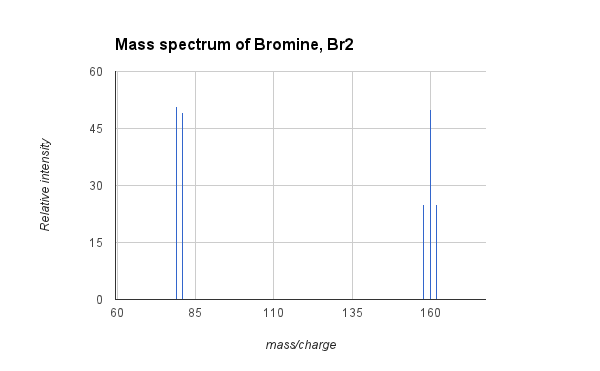Enduring Understanding 1.D: Experimental Data and Atomic Structure

• The current model of the atom is based on quantum mechanics (QM) and Coulomb's Law.
• QM predicts that electrons exist in regions of space called orbitals, and no more than two electrons can be in a single orbital. If two electrons are in an orbital, they must have opposite spin.
• An early model of the atom (Dalton's model) predicted that all atoms of the same element must be identical.
• However, experimental evidence obtained by Mass Spectrometry (MS) showed that this is not correct.
• In MS, samples of atoms or molecules are vaporized and ionized in a magnetic field. The gaseous ion curves through the magnetic field, and the degree of the curvature gives information about the charge and the mass of the ion.
• Example: Mass spectrum of Bromine, Br2:
•• Isotopes have the same number of protons but different number of neutrons. Every element has a characteristic relative abundance of its isotopes.
• The graphic above shows the mass spectrum of bromine gas, Br2. Natural bromine consists of two isotopes of bromine, at a nearly equal abundance, with atomic masses of 79 and 81. Molecular bromine (Br2) can therefore be composed (25% probability) of two atoms of 79Br and have a mass of 158, one atom of 79Br and one of 81Br (50% probability) with a mass of 160, or two atoms of 81Br (25% probability) with a mass of 162. The MS above shows the signals for the three peaks corresponding to the three isotopic compositions of Br2, and also the peaks from fragmentation to a bromine cation at 79 and 81. The average atomic mass of bromine is 79.9, which is the weighted average of the masses of the two isotopes.
• The structure of atoms and molecules can be probed by examining light energy (photons) that is absorbed or emitted by the atom or molecule. This is called spectroscopy.
• Photons of light have different energies based on their frequency, according to Planck's equation: E=hv.
• Absorption and emission of different wavelengths results from different kinds of molecular motion:
• Infrared photons represent changes in molecular vibrations. This can be useful for the detection of organic function groups, like alcohols (-OH) and ketones (C = O)
• Visible and ultraviolet photons represent transitions of valence electrons between energy levels.
• X-rays can result in ejection of core electrons (see photoelectron spectroscopy)
• Molecules absorb light to a degree proportional to their concentration. This means that the concentration of a molecule can be determined using Beer's Law: A = εbc, where A Is the absorbance, ε is the molar absorptivity of the molecule, b is the path length, and c is the concentration.
• UV/V is spectroscopy is especially useful for measuring the concentration of colored species in solution.

• Example. Gas A absorbs light at 440 nm and is orange in color. Gas B does not absorb at 440 nm and is colorless. Which of the following can we conclude about A and B? A has more vibrational modes than B, A has a lower first ionization energy than B, or A has lower energy electron transitions than B?
• We can conclude that A has lower energy electron transitions than B. Visible light spectroscopy involves electron energy level transitions, not vibrations (infrared spectroscopy) or ionizations (photoelectron spectroscopy).

 Related Links: Chemistry Chemistry Quizzes AP Chemistry Notes Periodicity AP Chemistry Quizzes Atoms, Molecules, and Chemical Analysis Quiz Electronic Structure of Atoms Quiz Periodicity, Atomic & Ionic Radii, Atomic Models Quiz Conservation of Matter and Balanced Equations Quiz Gases and Solutions Quiz Intermolecular Forces Quiz Molecular Geometry and VSEPR Quiz Bonding and Solid State Properties Quiz Balanced and Ionic Equations Quiz Chemical Reactions Quiz Redox Reactions and Electrochemistry Quiz Chemical Kinetics and Rates Quiz Reaction Rates and Collisions Quiz Multi-Step Reaction Rates and Catalysis Quiz Energy and Heat Quiz Conservation of Energy and Calorimetry Quiz Bond Energies Quiz Entropy and Gibbs Free Energy Quiz Chemical Equilibrium Quiz Solubility and Acid-Base Equilibrium Quiz

To link to this Experimental Data and Atomic Structure page, copy the following code to your site: